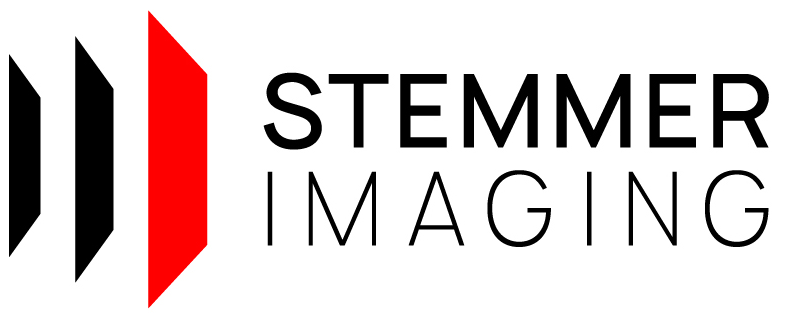CVB++ 14.0
Foundation/CppMetricCalibrationInclinationLaserPlane
1// ---------------------------------------------------------------------------
17// ---------------------------------------------------------------------------
18
19
20#include <iostream>
21
22#include "cvb/point_cloud_factory.hpp"
23#include "cvb/calibrator_3d.hpp"
24
25#include "cvb/foundation/metric_aqs12.hpp"
26
27
29void PrintTrafo(const Cvb::AffineMatrix3D& trafo);
30void PrintResiduals(const std::array<Cvb::Point3D<double>, 12>& residuals);
31void PrintAqs12Points(const std::vector< Cvb::Point3D<double>>& points);
32bool CheckAccuracy(const std::array<Cvb::Point3D<double>, 12>& residuals, double desiredAccuracy);
33
34int main()
35{
36 try
37 {
38 std::cout << "Estimation of an affine transformation (correcting an inclined laser plane)\n\n";
39
40 // load range map of AQS12
41 const auto rangemapFile = Cvb::InstallPath() + CVB_LIT("tutorial/Metric/Images/RangeMapCalibrationPattern.tif");
43 std::cout << "Rangemap loaded with size of " << rangemap->Width() << " x " << rangemap->Height() << " from " << rangemapFile << ".\n";
44
45 // create calibration configuration object
46 auto aqs12 = GetAqs12();
48 config->SetHomographyCalculated(false);
49
50 // create calibrated (dense) point cloud
51 const auto calibrationFile = Cvb::InstallPath() + CVB_LIT("tutorial/Metric/Images/SICalibration.json");
53 calibrator->SetRangeMapIgnoreValue(0.0);
54 auto cloud = Cvb::PointCloudFactory::Create<Cvb::DensePointCloud>(rangemap->Plane(0), *calibrator, Cvb::PointCloudFlags::Float | Cvb::PointCloudFlags::XYZConfidence);
55 std::cout << "Dense point cloud created from rangemap and calibration file with " << cloud->NumPoints() << " points.\n\n";
56
57 // create AQS12 segmentor for dense point clouds
59
60 // estimate calibration parameters
61 Cvb::AffineMatrix3D affineTrafo;
63 std::tie(affineTrafo,residuals) = Cvb::Foundation::Metric::CalculateCorrectionOfLaserPlaneInclinationFromAqs12Piece(*cloud, *segmentor, *config);
64 calibrator->SetCorrectionOfLaserPlaneInclination(affineTrafo);
65
66 // show results
67 PrintTrafo(affineTrafo);
68 PrintResiduals(residuals);
69
70 // check intermediate results if residuals are not ok
71 double desiredAccuracy = 0.05;
72 if (!CheckAccuracy(residuals, desiredAccuracy))
73 {
74 std::cout << "Results do not have desired accuracy. Check face segmentation and extracted AQS12 points...\n";
75 auto facesAqs12 = segmentor->FaceSegmentationFromPiece(*cloud);
76 auto pointsAqs12 = segmentor->ExtractProjectedPointsFromPiece(*cloud);
77 PrintAqs12Points(pointsAqs12);
78 }
79 else
80 {
81 // create calibrated cloud
82 auto calibratedCloud = Cvb::PointCloudFactory::Create<Cvb::DensePointCloud>(rangemap->Plane(0), *calibrator, Cvb::PointCloudFlags::Float | Cvb::PointCloudFlags::XYZConfidence);
83 std::cout << "The calibration was sucessful and accuracy is < " << desiredAccuracy << " mm. :)\n";
84 std::cout << "Lattice size of calibrated cloud: " << calibratedCloud->LatticeSize().Width() << " x " << calibratedCloud->LatticeSize().Height() << "\n";
85 }
86 }
87 catch (const std::exception & error)
88 {
89 std::cout << error.what() << std::endl;
90 }
91
92 return 0;
93}
94
96{
97 // reference points in mm in a right-handed coordindate system
99 { {
100 {20.0018, 44.9941, 15.0000},
101 {24.0018, 39.9942, 14.9994},
102 {23.9994, 24.9972, 15.0001},
103 {20.0021, 20.0035, 15.0011},
104 {15.9994, 25.0079, 15.0016},
105 {16.0000, 39.9919, 15.0010},
106 {20.0095, 59.9985, 4.9902},
107 {32.0093, 44.9958, 4.9909},
108 {32.0052, 19.9925, 4.9920},
109 {20.0021, 4.9961, 4.9939},
110 { 8.0024, 19.9980, 5.0009},
111 { 8.0065, 45.0009, 4.9984},
112 } };
113
115}
116
117bool CheckAccuracy(const std::array<Cvb::Point3D<double>, 12>& residuals, double desiredAccuracy)
118{
119 for (auto residual : residuals)
120 {
121 if (std::abs(residual.X()) > desiredAccuracy || std::abs(residual.Y()) > desiredAccuracy || std::abs(residual.Z()) > desiredAccuracy)
122 {
123 return false;
124 }
125 }
126 return true;
127}
128
129void PrintTrafo(const Cvb::AffineMatrix3D& trafo)
130{
131 std::cout << "Estimated transformation:\n";
132 std::cout << "translation: [";
133 std::cout << trafo.Translation().X() << ", " << trafo.Translation().Y() << ", " << trafo.Translation().Z() << "]\n";
134
135 std::cout << "transformation matrix: [\n";
136 std::cout << trafo.Matrix() << ", " << trafo.Matrix() << ", " << trafo.Matrix() << ",\n";
137 std::cout << trafo.Matrix() << ", " << trafo.Matrix() << ", " << trafo.Matrix() << ",\n";
138 std::cout << trafo.Matrix() << ", " << trafo.Matrix() << ", " << trafo.Matrix() << "]\n\n";
139}
140
141void PrintResiduals(const std::array<Cvb::Point3D<double>, 12>& residuals)
142{
143 std::cout << "Residuals:\n";
144 for (auto residual : residuals)
145 {
146 std::cout << residual.X() << " " << residual.Y() << " " << residual.Z() << "\n";
147 }
148 std::cout << "\n";
149}
150
151void PrintAqs12Points(const std::vector< Cvb::Point3D<double>>& points)
152{
153 std::cout << "AQS12 points\n";
154 for (auto point: points)
155 {
156 std::cout << point.X() << " " << point.Y() << " " << point.Z() << "\n";
157 }
158 std::cout << "\n";
159}
Affine transformation matrix for 3D.
Definition: affine_matrix_3d.hpp:98
Vector3D< double > Translation() const noexcept
Gets the translation part of the transformation.
Definition: affine_matrix_3d.hpp:157
Matrix3D Matrix() const noexcept
Gets the matrix part of the transformation.
Definition: affine_matrix_3d.hpp:137
Object to collect all input parameters for the AQS12 calibration piece.
Definition: decl_metric_aqs12_calibration_piece.hpp:28
static std::shared_ptr< AQS12DensePointCloudSegmentor > Create(const SegmentationMethod method)
Creates a AQS12 segmentor for dense point clouds based on given segmentation method.
Definition: decl_metric_segmentor_dense_point_cloud_aqs12.hpp:38
static std::unique_ptr< CalibrationConfiguration > Create(const AQS12Piece &aqs12)
Creates a calibration configuration object.
Definition: decl_metric_calibration_configuration.hpp:51
static std::unique_ptr< Image > Load(const String &fileName)
Loads an image with the given file name.
Definition: detail_image.hpp:32
AQS12TransformationResult CalculateCorrectionOfLaserPlaneInclinationFromAqs12Piece(const DensePointCloud &cloud, const AQS12DensePointCloudSegmentor &segmentor, const CalibrationConfiguration &config)
Calculates the correction of the laser plane inclination (affine transformation) from the given dense...
Definition: metric_aqs12.hpp:134
@ KmeansClustering
Clustering top, base and faces using kmeans.